# Examine Each Pair of Triangles in Figure, and State Which Pair of Triangles Are Similar. Also, State the Similarity Criterion Used by You for Answering - Mathematics

Sum

Examine each pair of triangles in Figure, and state which pair of triangles are similar. Also, state the similarity criterion used by you for answering the question and write the similarity relation in symbolic form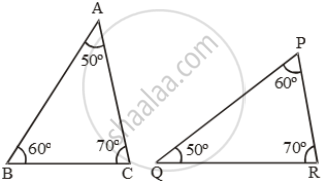figure (i)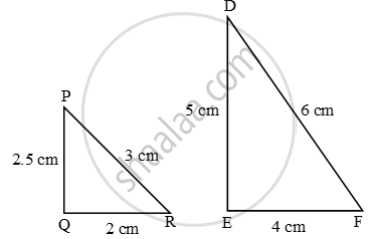figure 2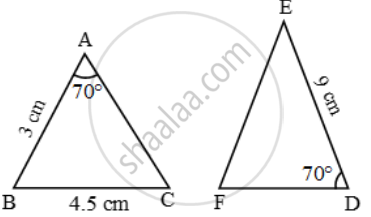figure 3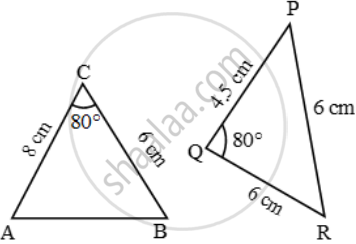figure 4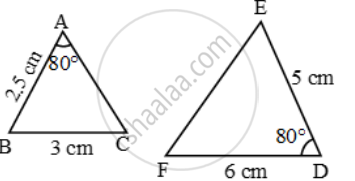figure 5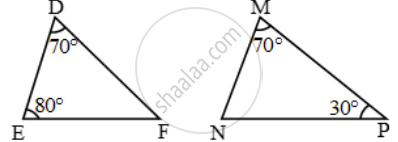figure 6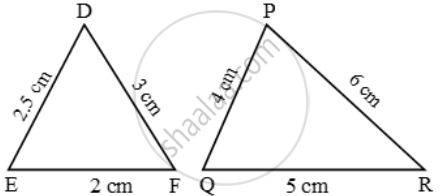figure 7

#### Solution

(i) ∠A = ∠Q, ∠B = ∠P and ∠C = ∠R.

∴ ∆ABC ~ ∆QPR (AAA-similarity)

(ii) In triangle PQR and DEF, we observe that

\frac{PQ}{DE}=\frac{QR}{EF}=\frac{PR}{DF}=\frac{1}{2}

Therefore, by SSS-criterion of similarity, we have

∆PQR ~ ∆DEF

(iii) SAS-similarity is not satisfied as included angles are not equal.

(iv) ∆CAB ~ ∆QRP (SAS-similarity), as

\frac{CA}{QR}=\frac{CB}{QP} and ∠C = ∠Q.

(v) In ∆’s ABC and DEF, we have

∠A = ∠D = 80º

\text{But, }\frac{AB}{DE}\ne \frac{AC}{DF} [∵ AC is not given]

So, by SAS-criterion of similarity these two triangles are not similar.

(vi) In ∆’s DEF and MNP, we have

∠D = ∠M = 70º

∠E = ∠N = 80º [∵ ∠N = 180º – ∠M – ∠P = 180º – 70º – 30º = 80º]

So, by AA-criterion of similarity

∆DEF ~ ∆MNP.

(vii) FE = 2 cm, FD = 3 cm, ED = 2.5 cm

PQ = 4 cm, PR = 6 cm, QR = 5 cm

∴ ∆FED ~ ∆PQR (SSS-similarity)

Concept: Similarity
Is there an error in this question or solution?